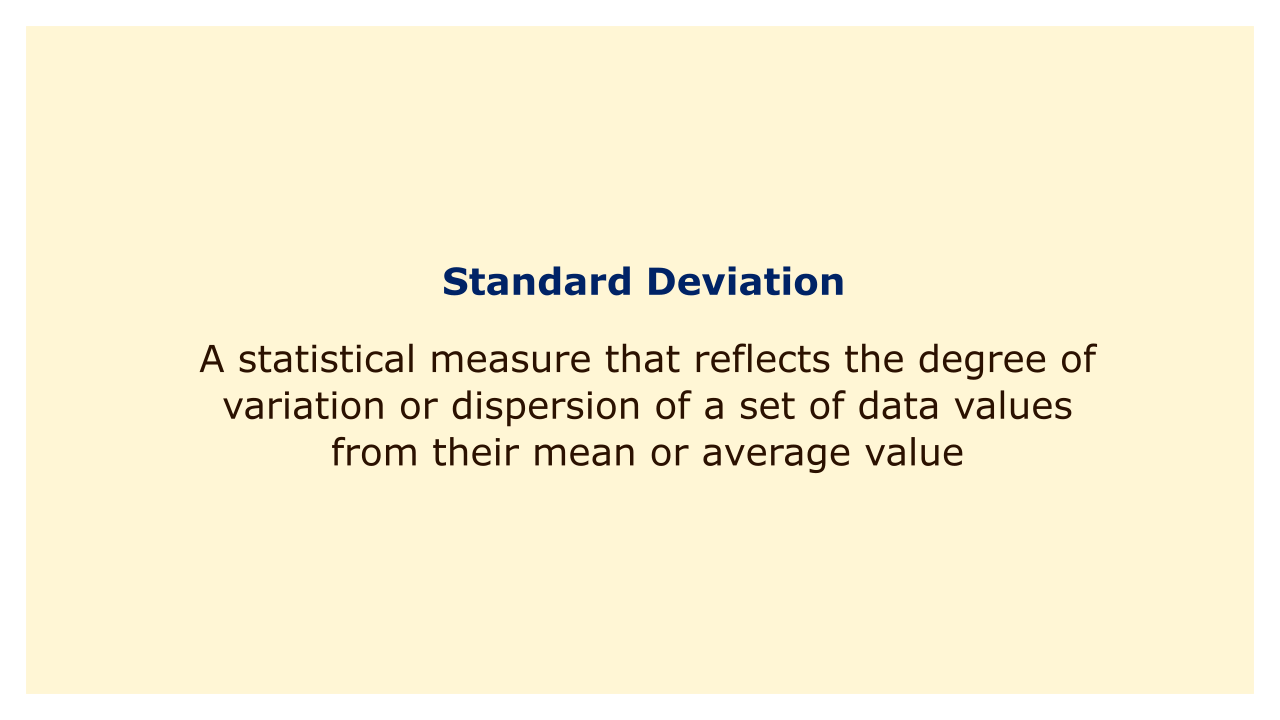# Standard DeviationImage: Moneybestpal.com

### A statistical measure called standard deviation expresses how widely distributed or how much a group of data values deviate from their mean or average value. Among other uses, it is frequently used in finance to evaluate the risk associated with a portfolio of investments.

The standard deviation of returns is a crucial risk indicator in finance since it shows how volatile or unpredictable returns are. A lower standard deviation denotes less risk and greater predictability, while a higher standard deviation denotes greater uncertainty and risk.

The standard deviation is calculated as the square root of the variance of the data set, where variance is the average of the squared deviations of each data point from the mean. This means that a data set with a larger range of values or more variability will have a higher standard deviation, and vice versa.

Standard deviation is a measurement that financial analysts and investors use to analyze the risk and return characteristics of various investments, such as stocks or mutual funds. For instance, in comparison to a stock with a smaller standard deviation, a stock with a high standard deviation may offer the possibility of higher returns, but also greater risk.
Tags